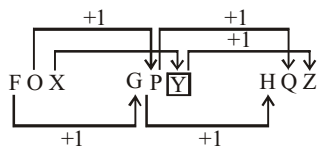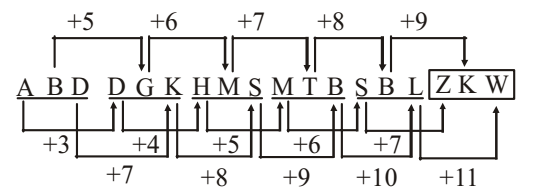## Number Series

#### Number Series

Direction: Read the given series and complete this series.

1. FOX, GP ?, HQZ
1. Y
2. Z
3. T
4. W
5. None of these

1. As per the details in the question the series is in the form of
From given figure , it is clear that we are getting next all others terms of given series on adding 1 in every previous alphabet of series .

##### Correct Option: A

As per the details in the question the series is in the form of
From given figure , it is clear that we are getting next all others terms of given series on adding 1 in every previous alphabet of series .
Hence the place value of ? is Y .1. YEB, WFD, UHG, SKI, (?)
1. QOL
2. TOL
3. QGL
4. QNL
5. None of these

1. As per the details in the question the series is in the form of
1st letter moves –2 steps each time.
2nd letter moves +1, +2, +3, +4 steps respectively.
3rd letter moves +2, +3, steps alternatively.

##### Correct Option: A

As per the details in the question the series is in the form of
1st letter moves –2 steps each time.
2nd letter moves +1, +2, +3, +4 steps respectively.
3rd letter moves +2, +3, steps alternatively.
Hence the required answer is QOL .

1. BEH, KNQ, TWZ, ?
1. IJL
2. CFI
3. BDF
5. None of these

1. All the letters of each term are moved nine steps forward to obtain the corresponding letters of the next term.

##### Correct Option: B

As per the details in the question the series is in the form of
All the letters of each term are moved nine steps forward to obtain the corresponding letters of the next term.
So , the next term of given series will be CFI .

1. A, CD, GHI, ?, UVWXY
1. LMNO
2. MNO
3. NOPQ
4. MNOP
5. None of these

1. The number of letters in the terms goes on increasing by one at each step. Also, there is a gap of one letter between the last letter of the first term and first letter of the second term and a gap of two letters between the last letter of the second term and first letter of the third term. So, the first letter of the required term would be four steps ahead of the last letter of the third term.

##### Correct Option: D

The number of letters in the terms goes on increasing by one at each step. Also, there is a gap of one letter between the last letter of the first term and first letter of the second term and a gap of two letters between the last letter of the second term and first letter of the third term. So, the first letter of the required term would be four steps ahead of the last letter of the third term.
The order of above series given as -
A, CD, GHI , ( ? ) , UVWXY
1 , 3 4 , 7 8 9 , ( 13 14 15 16 ) , 21 22 23 24 25
So ? = MNOP → 13 14 15 16
Hence , required answer will be MNOP .

1. ABD, DGK, HMS, MTB, SBL, ?
1. ZAB
2. XKW
3. ZKU
4. ZKW
5. None of these

1. As per the details in the question the series is in the form of
From given figure , it is clear that
Hence the place value of ? is ZKW .

##### Correct Option: D

As per the details in the question the series is in the form of
From given figure , it is clear that
Hence the place value of ? is ZKW .